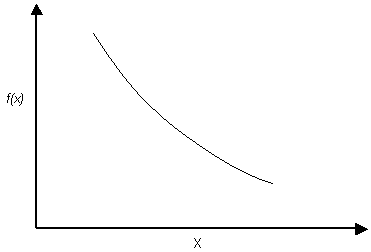# Exponential Distribution

The Exponential probability density function is available in RocPlane:Exponential probability density function.

NOTES:

• The range of values must always be positive for an Exponential Distribution. It must therefore not be used for orientation distributions which include negative values (e.g. Dip values less than zero).
• The mean of an Exponential Distribution is always equal to its standard deviation. This is a property of the Exponential Distribution and cannot be altered by the user.
• Like the Normal Distribution, the Exponential Distribution can be truncated by entering the desired minimum and maximum values (the basic Exponential Distribution varies from zero to infinity).
• The Exponential Distribution is sometimes used to define events, such as the occurrence of earthquakes or rockbursts, or quantities, such as the length of joints in a rock mass. Of the currently defined statistical variables in RocPlane, you may occasionally find it useful for modeling joint cohesion, for example.